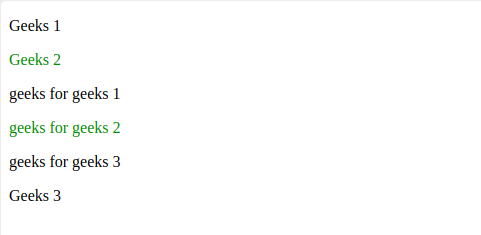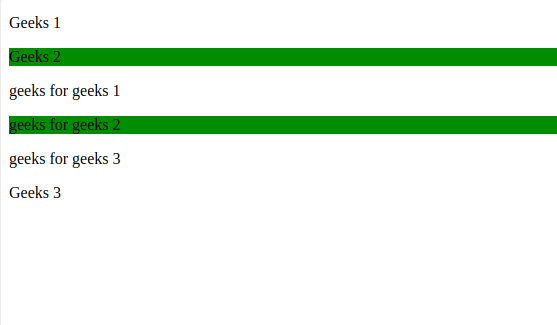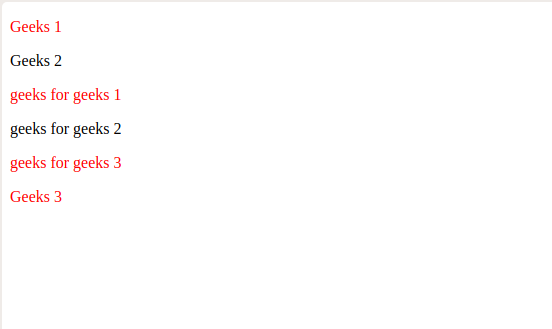# jQuery | :nth-of-type() Selector

The :nth-of-type() is an inbuilt selector in jQuery, used to select all the nth-child elements of specified parent.

Syntax :

``` parent_name : nth-of-type(n|even|odd|algebric equation)
```

Parameters: It takes a parameter n | even | odd | algebric equation.

Value Description
n Select the child present at nth index (starting from 1). n must be an integer.
even Selects the child present at even index.
odd Selects the child present at odd index.
algebric equation Select the child present at the value of the equation, equation should be of type mn + c or mn – c, where m and c are constant values.

Note:

• Child elements in different sections or divisions treated differently
i.e, indexing starts from beginning.
• In mn + c, n is starting from value 0.

Example-1: Using n as a parameter.

 ` ` `<``html``> ` ` `  `<``head``> ` `    ``<``script` `src``= ` `"https://ajax.googleapis.com/ajax/libs/jquery/3.3.1/jquery.min.js"``> ` `  `` ` `   `  `  ``<``script``> ` `        ``\$(document).ready(function() { ` `            ``\$("p:nth-of-type(2)").css( ` `              ``"color", "green"); ` `        ``}); ` `    `` ` ` ` ` `  `<``body``> ` `    ``<``p``>Geeks 1 ` `    ``<``p``>Geeks 2 ` ` `  `    ``<``section``> ` ` `  `    ``` ` `  `        ``<``p``>geeks for geeks 1 ` `        ``<``p``>geeks for geeks 2 ` `        ``<``p``>geeks for geeks 3 ` `    `` ` ` `  `    ``` `    ``<``p``>Geeks 3 ` ` `  ` ` ` `  ` `

Output:In the above example, child element at index 2 (parent is p tag) formatted into green color i.e. “Geeks 2” and “geeks for geeks 2”.

Example-2: Using even as a parameter.

 ` ` `<``html``> ` ` `  `<``head``> ` `    ``<``script` `src``= ` `"https://ajax.googleapis.com/ajax/libs/jquery/3.3.1/jquery.min.js"``> ` `  `` ` `  `  `  ``<``script``> ` `        ``\$(document).mouseover(function() { ` `            ``\$("p:nth-of-type(even)").css( ` `              ``"background-color", "green"); ` `        ``}); ` `    `` ` ` ` ` `  `<``body``> ` `    ``<``p``>Geeks 1 ` `    ``<``p``>Geeks 2 ` ` `  `    ``<``section``> ` `        ``` `        ``<``p``>geeks for geeks 1 ` `        ``<``p``>geeks for geeks 2 ` `        ``<``p``>geeks for geeks 3 ` `    `` ` ` `  `    ``` `    ``<``p``>Geeks 3 ` ` `  ` ` ` `  ` `

Output:In the above example, child element at even indices (parent is p tag) formatted into green color background i.e. “Geeks 2” and “geeks for geeks 2”.

Example-3: Using odd as a parameter.

 ` ` `<``html``> ` ` `  `<``head``> ` `    ``<``script` `src``= ` `"https://ajax.googleapis.com/ajax/libs/jquery/3.3.1/jquery.min.js"``> ` `  `` ` `    `  `  ``<``script``> ` `        ``\$(document).mouseover(function() { ` `            ``\$("p:nth-of-type(odd)").css( ` `              ``"color", "red"); ` `        ``}); ` `    `` ` ` ` ` `  `<``body``> ` `    ``<``p``>Geeks 1 ` `    ``<``p``>Geeks 2 ` ` `  `    ``<``section``> ` `        ``` `        ``<``p``>geeks for geeks 1 ` `        ``<``p``>geeks for geeks 2 ` `        ``<``p``>geeks for geeks 3 ` `    `` ` ` `  `    ``` `    ``<``p``>Geeks 3 ` ` `  ` ` ` `  ` `

Output:In the above example, child element at odd indices (parent is p tag) formatted into red colour i.e. “Geeks 1”, “geeks for geeks 1”, “geeks for geeks 3” and “Geeks 3”.

Example-4: Using algebric equation as a parameter.

 ` ` `<``html``> ` ` `  `<``head``> ` `    ``<``script` `src``= ` `"https://ajax.googleapis.com/ajax/libs/jquery/3.3.1/jquery.min.js"``> ` `  `` ` `   `  `  ``<``script``> ` `        ``\$(document).mouseover(function() { ` `            ``\$("p:nth-of-type(3n+2)").css( ` `              ``"color", "green"); ` `        ``}); ` `    `` ` ` ` ` `  `<``body``> ` `    ``<``p``>Geeks 1 ` `    ``<``p``>Geeks 2 ` ` `  `    ``<``section``> ` `        ``` `        ``<``p``>geeks for geeks 1 ` `        ``<``p``>geeks for geeks 2 ` `        ``<``p``>geeks for geeks 3 ` `        ``<``p``>geeks for geeks 4 ` `        ``<``p``>geeks for geeks 5 ` `    `` ` ` `  `    ``` `    ``<``p``>Geeks 3 ` ` `  ` ` ` `  ` `

Output:In the above example, child element at indices value equal to 3n + 2 (parent is p tag) formatted into green colour i.e. “Geeks 2”, “geeks for geeks 2”, “geeks for geeks 5”.

My Personal Notes arrow_drop_upCheck out this Author's contributed articles.

If you like GeeksforGeeks and would like to contribute, you can also write an article using contribute.geeksforgeeks.org or mail your article to contribute@geeksforgeeks.org. See your article appearing on the GeeksforGeeks main page and help other Geeks.

Please Improve this article if you find anything incorrect by clicking on the "Improve Article" button below.

Article Tags :

2

Please write to us at contribute@geeksforgeeks.org to report any issue with the above content.